SBVS083E January   2007  – January 2015

PRODUCTION DATA.

1. Features
2. Applications
3. Description
4. Revision History
5. Pin Configuration and Functions
6. Specifications
7. Detailed Description
1. 7.1 Overview
2. 7.2 Functional Block Diagram
3. 7.3 Feature Description
4. 7.4 Device Functional Modes
5. 7.5 Programming
8. Application and Implementation
1. 8.1 Application Information
2. 8.2 Typical Application
1. 8.2.1 Design Requirements
2. 8.2.2 Detailed Design Procedure
3. 8.2.3 Application Curves
3. 8.3 Do's and Don'ts
9. Power Supply Recommendations
10. 10Layout
11. 11Device and Documentation Support
1. 11.1 Device Support
1. 11.1.1 Development Support
2. 11.1.2 Device Nomenclature
2. 11.2 Documentation Support
4. 11.4 Electrostatic Discharge Caution
5. 11.5 Glossary
12. 12Mechanical, Packaging, and Orderable Information

• DDC|5
• DRV|6
• DRV|6

6 Specifications

6.1 Absolute Maximum Ratings

over operating free-air temperature range (unless otherwise noted) (1)
MIN MAX UNIT
Voltage VIN –0.3 +6.0 V
VEN and VVSET –0.3 VIN + 0.3(2)
VOUT –0.3 VIN + 0.3
Current IOUT Internally limited
Output short-circuit duration Indefinite
Total continuous power dissipation, PDISS See Thermal Information
Temperature Operating junction, TJ –40 125 °C
Storage, Tstg –55 150 °C
(1) Stresses beyond those listed under Absolute Maximum Ratings may cause permanent damage to the device. These are stress ratings only, which do not imply functional operation of the device at these or any other conditions beyond those indicated under Recommended Operating Conditions. Exposure to absolute-maximum-rated conditions for extended periods may affect device reliability.
(2) VEN and VVSET absolute maximum rating are VIN + 0.3V or +6.0V, whichever is less.

6.2 ESD Ratings

VALUE UNIT
V(ESD) Electrostatic discharge Human body model (HBM), per ANSI/ESDA/JEDEC JS-001, all pins(1) ±2000 V
Charged device model (CDM), per JEDEC specification JESD22-C101, all pins(2) ±500
(1) JEDEC document JEP155 states that 500-V HBM allows safe manufacturing with a standard ESD control process.
(2) JEDEC document JEP157 states that 250-V CDM allows safe manufacturing with a standard ESD control process.

6.3 Recommended Operating Conditions

over operating junction temperature range (unless otherwise noted)
MIN NOM MAX UNIT
VIN Input voltage 2.2 5.5 V
VOUT Output voltage 1.8 4.2 V
VEN Enable voltage 0 VIN V
IOUT Output current 0 150 mA
TJ Junction temperature –40 125 °C

6.4 Thermal Information

THERMAL METRIC(1) TPS780xx UNIT
DDC DRV
5 PINS 6 PINS
RθJA Junction-to-ambient thermal resistance 193.0 65.9 °C/W
RθJC(top) Junction-to-case (top) thermal resistance 40.1 87.3
RθJB Junction-to-board thermal resistance 34.3 35.4
ψJT Junction-to-top characterization parameter 0.9 1.7
ψJB Junction-to-board characterization parameter 34.1 35.8
RθJC(bot) Junction-to-case (bottom) thermal resistance N/A 6.1

6.5 Electrical Characteristics

Over operating temperature range (TJ = –40°C to 125°C), VIN = VOUT(nom) + 0.5 V or 2.2 V, whichever is greater; IOUT = 100 μA, VVSET = VEN = VIN, COUT = 1.0 μF, fixed or adjustable, unless otherwise noted. Typical values at TJ = 25°C.
PARAMETER TEST CONDITIONS MIN TYP MAX UNIT
VIN Input voltage range 2.2 5.5 V
VOUT(1) DC output accuracy Nominal TJ = 25°C, VSET = high/low –2% ±1% +2%
Over VIN, IOUT, temperature VOUT(nom) + 0.5 V ≤ VIN ≤ 5.5 V,
0 mA ≤ IOUT ≤ 150 mA, VSET = high/low
–3.0% ±2.0% +3.0%
VFB Internal reference(9)
TJ = 25°C, VIN = 4.0 V, IOUT = 75 mA 1.216 V
VOUT_RANGE Output voltage range(2)(3)
VIN = 5.5 V, IOUT = 100 μA(9) VFB 5.25 V
ΔVOUT(ΔVIN) Line regulation VOUT(nom) + 0.5 V ≤ VIN ≤ 5.5 V,
IOUT = 5 mA
–1% +1%
ΔVOUT(ΔIOUT) Load regulation 0 mA ≤ IOUT ≤ 150 mA –2% +2%
VDO Dropout voltage(4) VIN = 95% VOUT(nom), IOUT = 150 mA 250 mV
Vn Output noise voltage BW = 100 Hz to 100 kHz, VIN = 2.2 V,
VOUT = 1.2 V, IOUT = 1 mA
86 μVRMS
VHI VSET high (output VOUT(LO) selected), or EN high (enabled) 1.2 VIN V
VLO VSET low (output VOUT(HI) selected), or EN low (disabled) 0 0.4 V
ICL Output current limit VOUT = 0.90 × VOUT(nom) 150 230 400 mA
IGND Ground pin current IOUT = 0 mA(5) 420 800 nA
IOUT = 150 mA 5 μA
ISHDN Shutdown current (IGND) VEN ≤ 0.4 V, 2.2 V ≤ VIN < 5.5 V,
TJ = –40°C to 100°C
18 130 nA
IVSET VSET pin current VEN = VVSET = 5.5 V 70 nA
IEN EN pin current VEN = VVSET = 5.5 V 40 nA
IFB FB pin current(10)
VIN = 5.5 V, VOUT = 1.2 V, IOUT = 100 μA 10 nA
PSRR Power-supply rejection ratio VIN = 4.3 V,
VOUT = 3.3 V,
IOUT = 150 mA
f = 10 Hz 40 dB
f = 100 Hz 20
f = 1kHz 15
tTR(H→L) VOUT transition time (high-to-low)
VOUT = 97% × VOUT(HI)
VOUT_LOW = 2.2 V, VOUT(HI) = 3.3 V,
IOUT = 10 mA
800 μs
tTR(L→H) VOUT transition time (low-to-high)
VOUT = 97% × VOUT(LO)
VOUT_HIGH = 3.3 V, VOUT(LO) = 2.2 V,
IOUT = 10 mA
800 μs
tSTR Start-up time(6) COUT = 1.0 μF, VOUT = 10% VOUT(nom) to VOUT = 90% VOUT(nom) 500 μs
tSHDN Shutdown time(7) IOUT = 150 mA, COUT = 1.0 μF,
VOUT = 2.8 V,
VOUT = 90% VOUT(nom) to
VOUT = 10% VOUT(nom)
500(8) μs
TSD Thermal shutdown temperature Shutdown, temperature increasing 160 °C
Reset, temperature decreasing 140 °C
TJ Operating junction temperature –40 125 °C
(1) The output voltage for VSET = low/high is programmed at the factory.
(2) No VSET pin on the adjustable version.
(3) No dynamic voltage scaling on the adjustable version.
(4) VDO is not measured for devices with VOUT(nom) < 2.3 V because minimum VIN = 2.2 V.
(5) IGND = 800 nA (max) up to 100°C.
(6) Time from VEN = 1.2 V to VOUT = 90% (VOUT(nom)).
(7) Time from VEN = 0.4 V to VOUT = 10% (VOUT(nom)).
(8) See Shutdown for more details.
(10) The TPS78001 FB pin is tied to VOUT. Adjustable version only.

6.6 Typical Characteristics

Over the operating temperature range of TJ = –40°C to 125°C, VIN = VOUT(nom) + 0.5 V or 2.2 V, whichever is greater; IOUT = 100 μA, VEN = VVSET = VIN, COUT = 1 μF, and CIN = 1 μF, unless otherwise noted.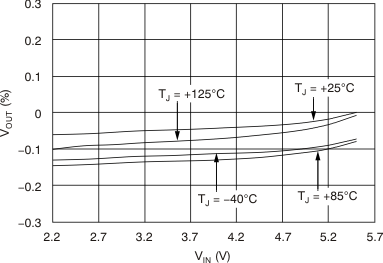IOUT = 5 mA VOUT(nom) = 1.22 V
Figure 1. TPS78001 Line Regulation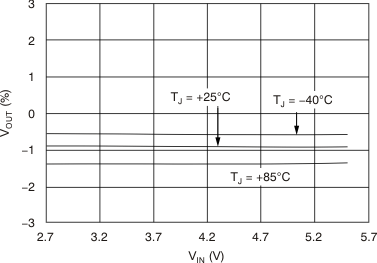IOUT = 150 mA VVSET = 1.2 V VOUT(nom) = 2.2 V
Figure 3. TPS780330220 Line Regulation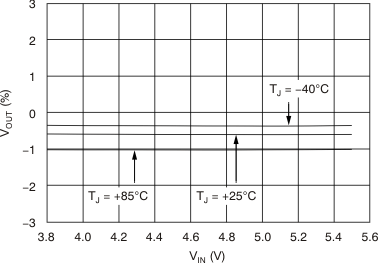IOUT = 150 mA VVSET = 0.4 V VOUT(nom) = 3.3 V
Figure 5. TPS780330220 Line Regulation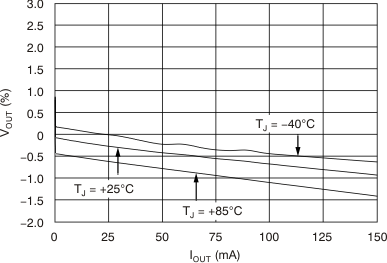VVSET = 1.2 V VIN = 2.7 V VOUT(nom) = 2.2 V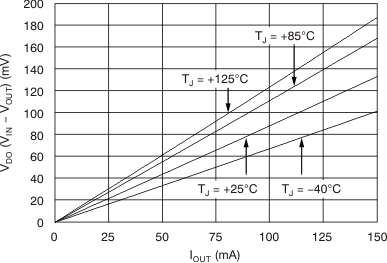VOUT(nom) = 3.3 V VIN = 0.95 × VOUT(nom)
Figure 9. TPS78001 Dropout Voltage vs Output Current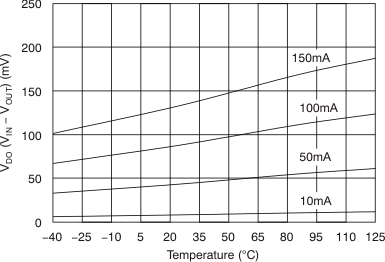VOUT(nom) = 3.3 VIN = 0.95 × VOUT(nom)
Figure 11. TPS78001 Dropout Voltage vs Temperature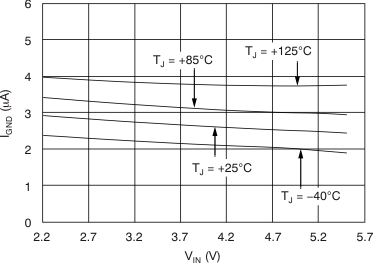IOUT = 50 mA VOUT(nom) = 1.22 V
Figure 13. TPS78001 Ground Pin Current vs Input VoltageIOUT = 0 mA VVSET = 1.2 V VOUT(nom) = 2.2 V
Figure 15. TPS780330220 Ground Pin Current vs Input VoltageIOUT = 50 mA VVSET = 1.2 V VOUT(nom) = 2.2 V
Figure 17. TPS780330220 Ground Pin Current vs Input Voltage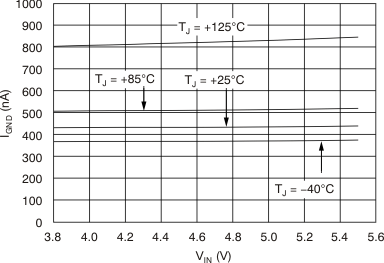IOUT = 0 mA VVSET = 0.4 V VOUT(nom) = 3.3 V
Figure 19. TPS780330220 Ground Pin Current vs Input Voltage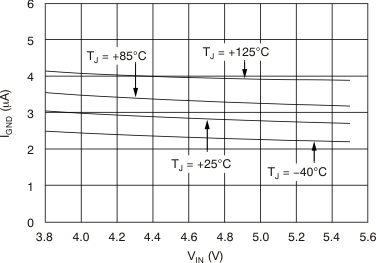IOUT = 50 mA VVSET = 0.4 V VOUT(nom) = 3.3 V
Figure 21. TPS780330220 Ground Pin Current vs Input Voltage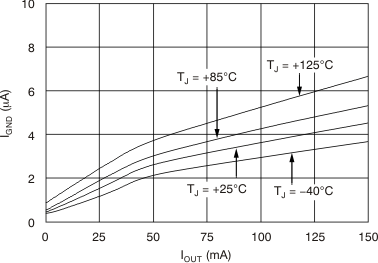VVSET = 1.2 V VIN = 5.5 V VOUT(nom) = 2.2 V
Figure 23. TPS780330220 Ground Pin Current vs Output Current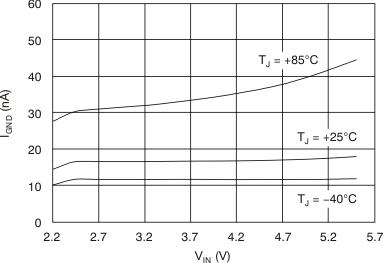IOUT = 0 mA VVSET = 0.4 V
Figure 25. TPS78001 Shutdown Current vs Input VoltageVVSET = 1.2 V VOUT = 95% VOUT(nom) VOUT(nom) = 2.2 V
Figure 27. TPS780330220 Current Limit vs Input VoltageIOUT = 0 mA VOUT(nom) = 1.22 V
Figure 29. TPS78001 Feedback Pin Current vs Temperature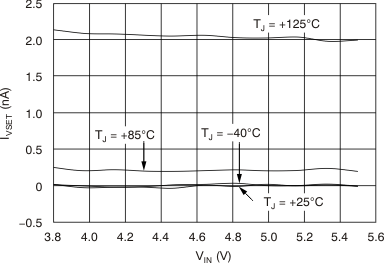IOUT = 100 μA VVSET = 0.4 V VOUT(nom) = 3.3 V
Figure 31. TPS780330220 VSET Pin Current vs Input Voltage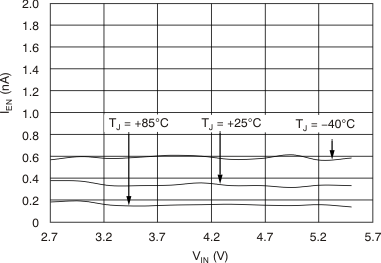IOUT = 100 μA VVSET = 1.2 V VOUT(nom) = 2.2 V
Figure 33. TPS780330220 Enable Pin Current vs Input Voltage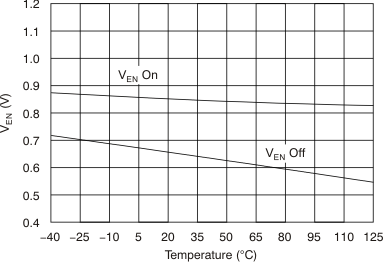IOUT = 1 mA
Figure 35. TPS78001 Enable Pin Hysteresis vs Temperature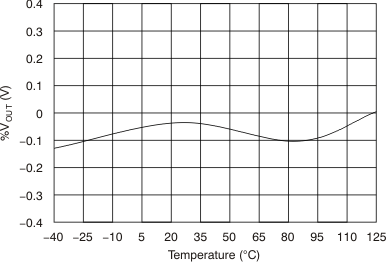IOUT = 1 mA VIN = 3.8 V VOUT(nom) = 3.3 V
Figure 37. TPS78001 %ΔVOUT vs Temperature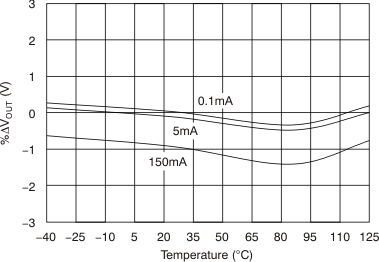VVSET = 0.4 V VIN = 3.8 V VOUT(nom) = 3.3 V
Figure 39. TPS780330220 %ΔVOUT vs Temperature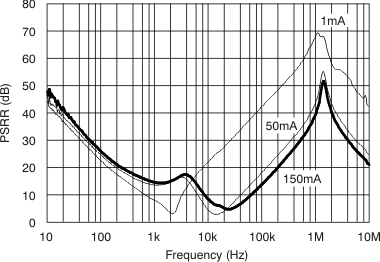VIN = 2.7 V VOUT = 1.2 V COUT = 2.2 μF
Figure 41. TPS78001 Ripple Rejection vs Frequency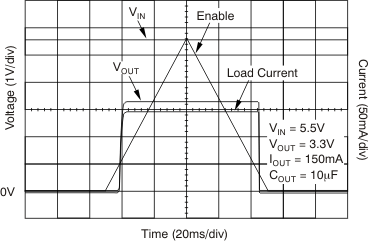Figure 43. TPS780330220 Output Voltage vs Enable (Slow Ramp)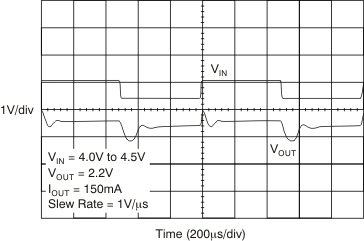Figure 45. TPS780330220 Line Transient Response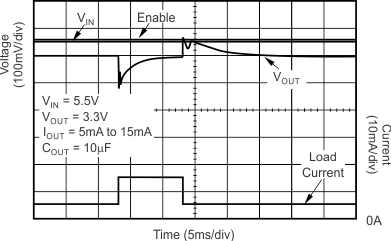Figure 47. TPS780330220 Load Transient ResponseFigure 49. TPS780330220 Enable Pin vs Output Voltage Response and Output Current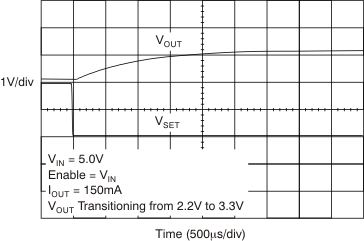Figure 51. TPS780330220 VSET Pin Toggle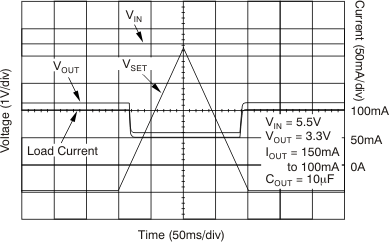Figure 53. TPS780330220 VSET Pin Toggle (Slow Ramp)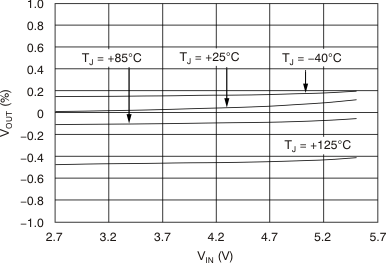IOUT = 5 mA VVSET = 1.2 V VOUT(nom) = 2.2 V
Figure 2. TPS780330220 Line Regulation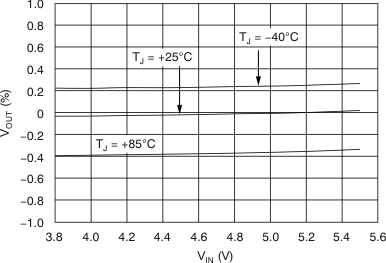IOUT = 5 mA VVSET = 0.4 V VOUT(nom) = 3.3 V
Figure 4. TPS780330220 Line Regulation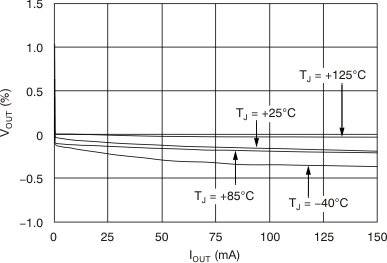VOUT(nom) = 3.3 V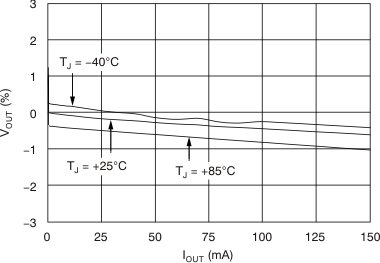VVSET = 0.4 V VIN = 3.8 V VOUT(nom) = 3.3 V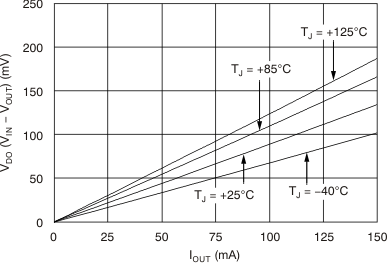VVSET = 0.4 V VOUT(nom) = 3.3 V VIN = 0.95 × VOUT(nom)
Figure 10. TPS780330220 Dropout Voltage vs Output Current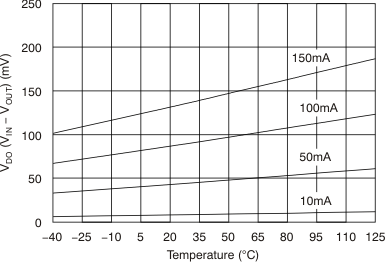VVSET = 0.4 V VOUT(nom) = 3.3 V VIN = 0.95 × VOUT(nom)
Figure 12. TPS780330220 Dropout Voltage vs Temperature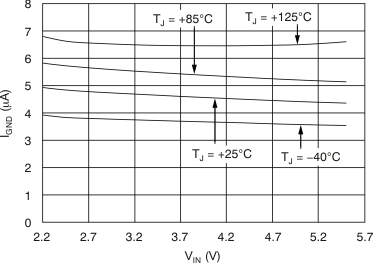IOUT = 150 mA VOUT(nom) = 1.22 V
Figure 14. TPS78001 Ground Pin Current vs Input VoltageIOUT = 1 mA VVSET = 1.2 V VOUT(nom) = 2.2 V
Figure 16. TPS780330220 Ground Pin Current vs Input Voltage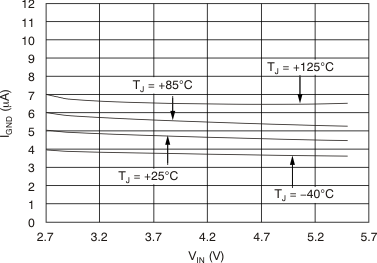IOUT = 150 mA VVSET = 1.2 V VOUT(nom) = 2.2 V
Figure 18. TPS780330220 Ground Pin Current vs Input Voltage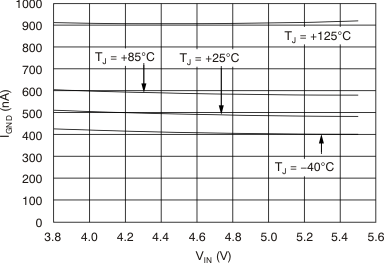IOUT = 1 mA VVSET = 0.4 V VOUT(nom) = 3.3 V
Figure 20. TPS780330220 Ground Pin Current vs Input Voltage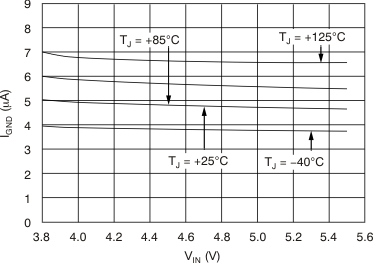IOUT = 150 mA VVSET = 0.4 V VOUT(nom) = 3.3 V
Figure 22. TPS780330220 Ground Pin Current vs Input Voltage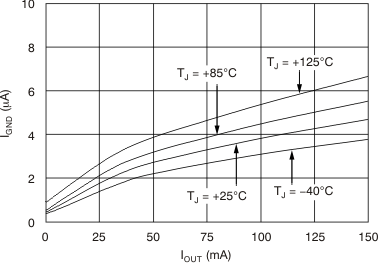VVSET = 0.4 V VIN = 5.5 V VOUT(nom) = 3.3 V
Figure 24. TPS780330220 Ground Pin Current vs Output Current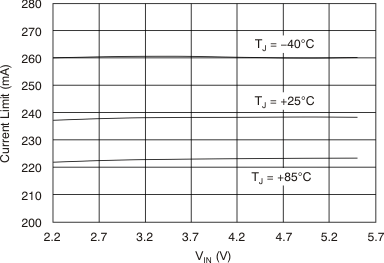VOUT = 90% VOUT(nom) VOUT(nom) = 1.22 V
Figure 26. TPS78001 Current Limit vs Input Voltage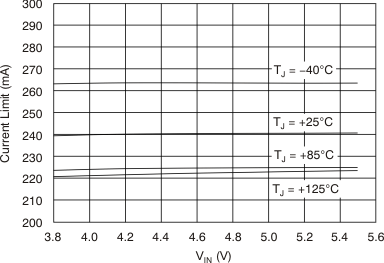VVSET = 0.4 V VOUT = 95% VOUT(nom) VOUT(nom) = 3.3 V
Figure 28. TPS780330220 Current Limit vs Input VoltageIOUT = 100 μA VVSET = 1.2 V VOUT(nom) = 2.2 V
Figure 30. TPS780330220 VSET Pin Current vs Input Voltage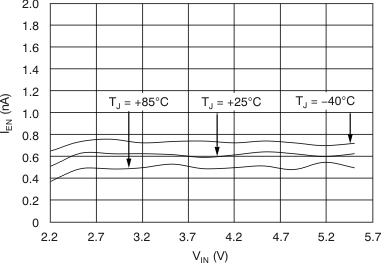IOUT = 1 mA VOUT(nom) = 1.22 V
Figure 32. TPS78001 Enable Pin Current vs Input Voltage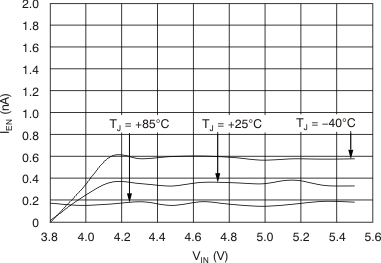IOUT = 100 μA VVSET = 0.4 V VOUT(nom) = 3.3 V
Figure 34. TPS780330220 Enable Pin Current vs Input Voltage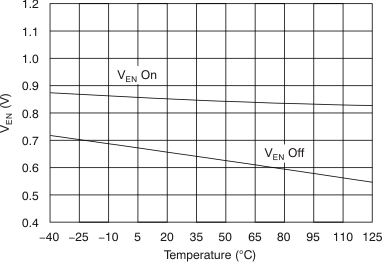IOUT = 1 mA
Figure 36. TPS780330220 Enable Pin Hysteresis vs Temperature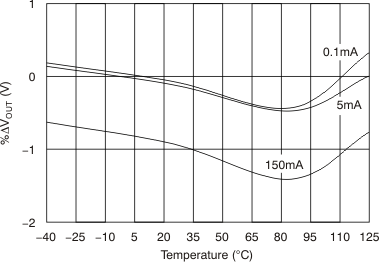VVSET = 1.2 V VIN = 2.7 V VOUT(nom) = 2.2 V
Figure 38. TPS780330220 %ΔVOUT vs Temperature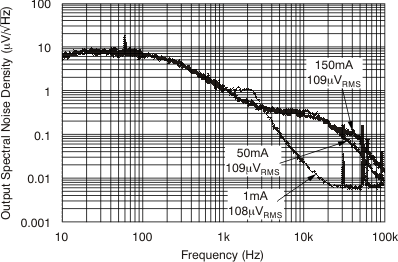CIN = 1 μF COUT = 2.2 μF VVSET = 1.2 V VIN = 2.7 V
Figure 40. TPS780330220 Output Spectral Noise Density vs Frequency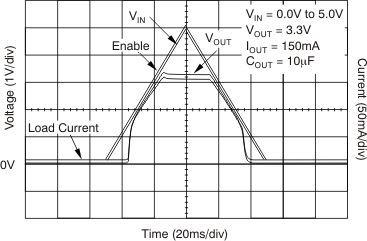Figure 42. TPS780330220 Input Voltage Ramp vs Output VoltageFigure 44. TPS780330220 Input Voltage vs Delay to Output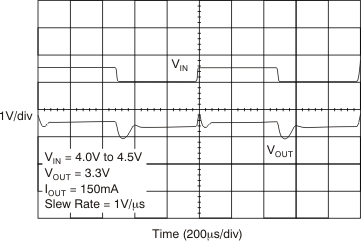Figure 46. TPS780330220 Line Transient ResponseFigure 48. TPS780330220 Load Transient Response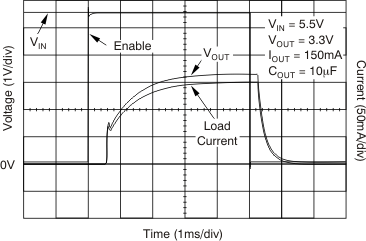Figure 50. TPS780330220 Enable Pin vs Output Voltage Delay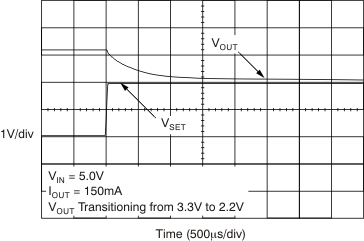Figure 52. TPS780330220 VSET Pin Toggle